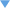Homepage Crypto Index Glossary Enigma Hagelin Fialka Rotor Pin-wheel Voice Data Hand OTP EMU HSM Mixers Phones Bulk FILL Codebooks Algorithms Cryptanalysis ToysCountries DDR Germany Netherlands Russia Switzerland UK USA USSR YugoslaviaManufacturers ANT Aristo Ascom AT&T BBC Bosch Compumatica Crypto AG Datotek Fox-IT GD Gemalto Gretag Harris HELL ITT Lorenz Lugagne MEL Mils Motorola Mykotronx Nokia OMI Omnisec Philips PTT R&S Racal RanData Raytheon RCA Safenet Secunet Siemens STK Tadiran Tait TCC Telefunken Telsy Teltron Thales Transvertex TST Ultra Electronics Utimaco Zellweger OTHER Spy radio Burst encoders Intercept Covert Radio PC Telex Telephones People Agencies Manufacturers DONATE Publications Standards For sale Kits Shop News Events Wanted Contact About us Links← SpellMaster
← Algorithm

C-code →1. SpellMaster in Python languageXavier Bonnetain — 29 July 2022 (9:13) The first one to solve the mystery is Xavier Bonnetain, a researcher in cryptography in the CARAMBA team at LORIA in Nancy (France). He made small changes to the recovered C-code and managed to reconstruct the alphabet substitution table entirely from the published PT/CT pairs. His solution is written in the Python programming language and can be downloaded below.DownloadSpellMaster algorithm in Python (ZIP) Xavier Bonnetain, 29 July 2022.Python code```   # Spellmaster encrypt/decrypt # Written by Xavier Bonnetain def e(c) : """[0,27] -> Alphabet""" if c < 26: return chr(c+ord('A')) if c == 26: return '-' return '?' def d(c) : """Alphabet -> [0,27]""" a = ord(c) if a <= ord('Z') and a >= ord('A'): return a - ord('A') if c == '-': return 26 return 27 def enc(key, message): """Encryption, post-permutation""" c = key s = "" for m in message: m = d(m) key = 0 for i in c: key = (key+ (i - 65)) if key < -128: key+=256 if key >= 128: key-=256 key = key %28 r = (key - m) % 28 s+=e(r) c= c[1:]+[(key+m)%28] return s def dec(key, message): """Decryption, pre-permutation""" c = key s = "" for m in message: m = d(m) key = 0 for i in c: key = (key+ (i - 65)) if key < -128: key+=256 if key >= 128: key-=256 key = key %28 r = (key - m) % 28 s+=e(r) c= c[1:]+[(key+r)%28] return s def getmatch(key, plaintext, ciphertext): """Compute the permutation from a pair""" c = key for i in range(len(ciphertext)): m = d(ciphertext[i]) key = 0 for j in c: key = (key+ (j - 65)) if key < -128: key+=256 key = key %28 r = (key - m) % 28 c= c[1:]+[(key+r)%28] print("'%c' : '%c' ," %(plaintext[i],e(r))) #The permutation dico = { 'A' : 'H' , 'B' : 'O' , 'C' : 'W' , 'D' : 'A' , 'E' : 'S' , 'F' : 'M' , 'G' : 'J' , 'H' : 'R' , 'I' : 'N' , 'J' : 'E' , 'K' : 'D' , 'L' : 'G' , 'M' : 'B' , 'N' : 'X' , 'O' : 'K' , 'P' : 'T' , 'Q' : 'C' , 'R' : 'Q' , 'S' : 'P' , 'T' : 'U' , 'U' : 'I' , 'V' : 'Y' , 'W' : 'F' , 'X' : 'Z' , 'Y' : 'L' , 'Z' : 'V', '-' : '-', '?' : '?' } def re(x): """Inverse permutation""" for d in dico: if dico[d] == x: return d raise AssertionError def expand(key): k =  * 8 for i in range(8): k[i] = key[i%len(key)] return k #####Toplevel functions def encrypt(key,message): m = [ dico[x] for x in message] k = [ d(x)+65 for x in key] return enc(expand(k),m) def decrypt(key,cipher): k = [ d(x)+65 for x in key] m = dec(expand(k),cipher) return ''.join(str(re(c)) for c in m) #####Tests def check(key,plain,cipher): s = encrypt(key,plain) print("Expected:\t"+cipher) print("Found:\t\t",end="") for (a,b) in zip(s,cipher): print(a,end="") print("\0") s = decrypt(key,cipher) print("Expected:\t"+plain) print("Found:\t\t",end="") for (a,b) in zip(s,plain): print(a,end="") print("\n") check("CATS","DOGSDOGSDOGSDOGS","WXCQ-DSUCEKQD-WU") check("CAT","DOGSDOGSDOGSDOGS","QLGAGVHPOCWEXCID") check("SECRECY","CRYPTOMUSEUM-FOREVER","LRCHZHE-GQBBSJROQ--R") check("SECRECY","LONG-LIVE-CRYPTOMUSEUM","?HIJVVI-XLXOKRKI?SKIQX") check("AAAAAAAA","AAAAAAAAAA","VTPL?DL?HV") check("AAAA","BBBB","OTBZ") check("AAAAAAAA","BBBBBBBBBBBBBBBB","OTBZNRZNVAJRJFVR") check("AAA","BBBBBBBBBBBBBBBBBBBBBBBBB","OTBZNRZNVAJRJFVRVVOTVBBJB") check("AAAAAAAA","BBBBBBBBBB","OTBZNRZNVA") check("AAAAAAAA","ABCDEFGHIJKLMNOPQRSTUVWXY","VMHTL?QFPCBKSZCJGRZM-EEEZ") check("AAAAAAAA","BCDEFGHIJKLMNOPQRSTUVWXYZ","OLXXXIRHMTOWV-JWBPCGQEIFY") check("AAAAAAAA","CDEFGHIJKLMNOPQRSTUVWXYZA","GN?HEJTIUVSJOV-ZLVFSUYZEH") check("AAAAAAAA","DEFGHIJKLMNOPQRSTUVWXYZAB","ABHIRHMYSZVONCRTFEJSUZAHA") check("AAAAAAAA","IS-SPELLMASTER-ALIVE?","PRQGBETNKDV?EHYE-RELK") check("BBBBBBBB","IS-SPELLMASTER-ALIVE?","XERHCFUOLZWAFIZF?SM-L") ```Further informationAny links shown in red are currently unavailable. If you like the information on this website, why not make a donation?
© Crypto Museum. Created: Friday 29 July 2022. Last changed: Wednesday, 03 August 2022 - 08:47 CET.